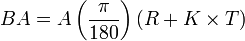# SOLIDWORKS Sheet Metal Bend Allowance CalculationsArticle by Andrew Lidstone, CSWE updated July 4, 2011

###### Article

In SOLIDWORKS there are several options for Sheet Metal Bend Allowance Calculations.  These calculations will determine the length of the flattened pattern shape.  Three of the most commonly used options are using a K-Factor value, a Bend Allowance value, or a Bend Deduction value.  Here are the calculations that SolidWorks is doing in the background when using these methods.

### K-Factor:

When you choose the K-Factor option SolidWorks will calculate a Bend Allowance (BA) value based on: the K-Factor you enter (K), the thickness of the material (T), the angle of the bend (A), and the inside radius of the bend (R).Once the BA is determined SolidWorks can calculate the flattened length of the bend using the method below.

### Bend Allowance:When you choose the Bend Allowance option you bypass SolidWorks’ calculating the BA value by entering it directly yourself.  The Bend Allowance value is the distance along the neutral axis of the bend, that is, a distance that remains unchanged when the part is folded or flattened.

The BA value can then be added to the flat distances (the distance from where the bend radius starts to the end of the part, or to the next bend radius) to determine the flattened length of the bend.  Adding all the flat distances of the part and the BA value of each bend will equal the flat pattern length.So for this part the flat pattern length would equal:(Total Flat Dimensions)+(Bend Allowance * Number of Bends) bought

( X+Y+Y+Z+Z)+(BA*4)

### Bend Deduction:

When you choose the Bend Deduction option SolidWorks will perform a much simpler operation to determine the flat pattern length.  The outside dimensions of the part are added together, then the Bend Deduction (BD) value you enter in is multiplied by the number of bends and subtracted from the total.So for this part the flat pattern length would equal:

(Total Outside Dimensions) – (Bend Deduction * Number Of Bends)

(A+B+B+C+C) – (BD*4)

For more information on these calculations please see Machinery Handbook Twenty Fifth Edition and McGraw-Hill Machining and Metalworking Handbook

#### Get Certified SOLIDWORKS Services from Javelin